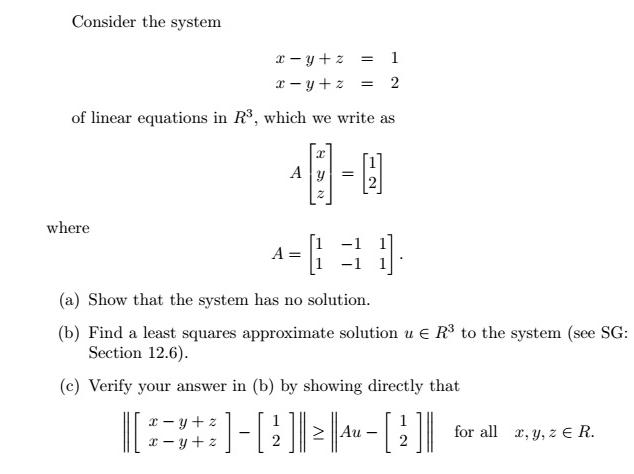# Least Squares Approximation

• Samuel Williams

#### Samuel Williams

Member warned about not using the HW template

My apologies for having to post in an image, my latex skills are not good enough for the question at hand :(

a) There is no solution since the system has more unknowns than equations (the equations are equal giving 1=2 which does not make sense).

b) I get a solution of \begin{bmatrix}1 \\1 \\ 3/2 \end{bmatrix}
for u.

c) I am not sure how to prove this directly, since there are no values for x,y or z.

First off, I do not see the picture for the problem, just the solution. Second, you should use the homework template in this forum.

My apologies for having to post in an image, my latex skills are not good enough for the question at hand :(

a) There is no solution since the system has more unknowns than equations (the equations are equal giving 1=2 which does not make sense).

b) I get a solution of \begin{bmatrix}1 \\1 \\ 3/2 \end{bmatrix}
for u.

c) I am not sure how to prove this directly, since there are no values for x,y or z.

If the problem is not very complicated to state, just type it out in plain text. LaTeX is great, but don't let not knowing it stand in your way.

Again, my apologies. It seems that I accidentally deleted the image.
So these are the questionsI did (a) and (b) as mentioned above and there are no relevant formulae or theorems that I am aware of for part (c).

Right. For part b, anything that lands you at x - y + z = 3/2 is correct.
For part c, you are asked to show that any other choice for x, y, z such that x-y+z is not equal to 3/2, (x-y+z - 1)^2 + (x-y+z-2)^2 > (3/2 - 1)^2 + (3/2-2)^2 and equal only when x-y+z = 3/2.
There are plenty of methods to attack the problem, but you should be able to make a good argument without referring to formulae or theorems.

•WWGD
Again, my apologies. It seems that I accidentally deleted the image.
So these are the questionsI did (a) and (b) as mentioned above and there are no relevant formulae or theorems that I am aware of for part (c).

For (c), Least Squares is supposed to minimize the norm as defined. I think it minimizes the L^2 -norm. Still, you have a reference there for SG section 12.6. Did you check it out?

Right. For part b, anything that lands you at x - y + z = 3/2 is correct.
For part c, you are asked to show that any other choice for x, y, z such that x-y+z is not equal to 3/2, (x-y+z - 1)^2 + (x-y+z-2)^2 > (3/2 - 1)^2 + (3/2-2)^2 and equal only when x-y+z = 3/2.
There are plenty of methods to attack the problem, but you should be able to make a good argument without referring to formulae or theorems.

Thanks, I managed to find a solution to the problem with your explanation to the question.

•RUber
Nice geometric approach, RUBER, recognizing that the "bisecting" plane is in the solution set, much simpler than anything I had in mind.

•RUber